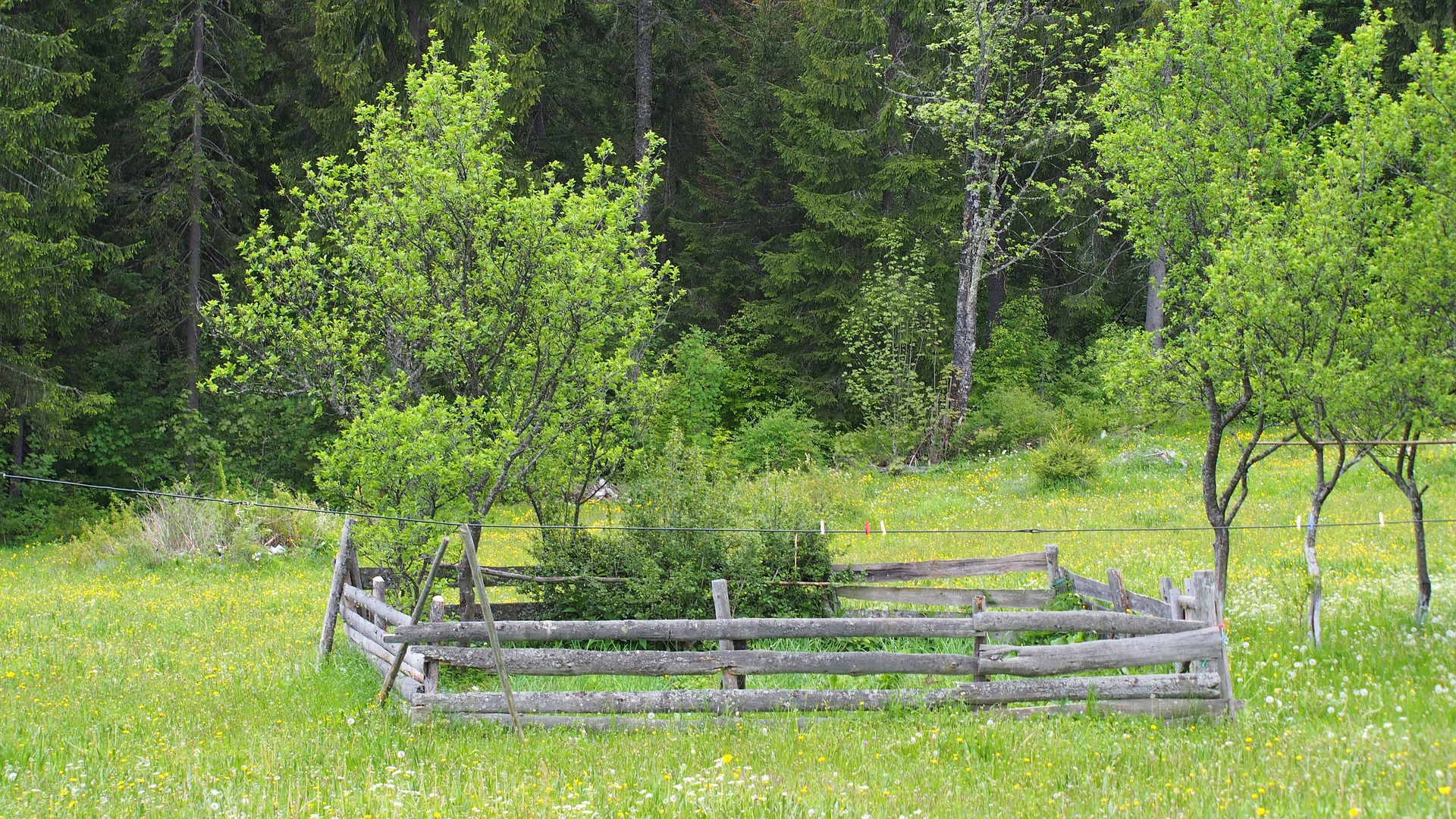Perimeter and AreaOutcomes

• Calculate the perimeter of a square, rectangle, and polygon
• Calculate the perimeter of a circle
• Calculate the area of a square, rectangle, and triangle
• Calculate the area of a circle and cylinder